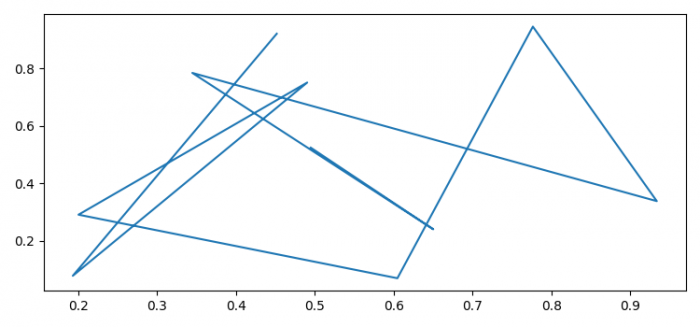# What is the difference between importing matplotlib and matplotlib.pyplot?

When we import matplotlib, we are importing all its libraries, whereas importing matplotlib.pyplot only imports pyplot's properties.

## steps

• Import matplotlib.pyplot as plt

• Set the figure size and adjust the padding between and around the subplots.

• Create x and y data points using numpy.

• Plot x and y data points using plot() method.

• To display the figure, use show() method.

## Example

import numpy as np
import matplotlib.pyplot as plt

plt.rcParams["figure.figsize"] = [7.50, 3.50]
plt.rcParams["figure.autolayout"] = True

x = np.random.rand(10)
y = np.random.rand(10)

plt.plot(x, y)

plt.show()

## OutputUpdated on: 10-Aug-2021

1K+ Views